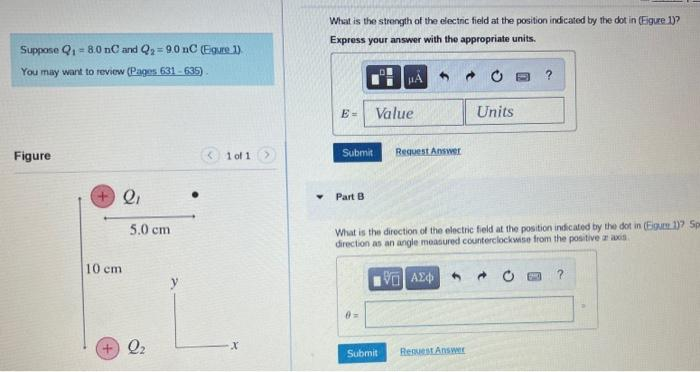Home / Expert Answers / Physics / what-is-the-strongth-of-the-electic-field-at-the-position-indicated-by-the-dot-in-eigure-1-suppo-pa219

# (Solved): What is the strongth of the electic field at the position indicated by the dot in (Eigure 1)? Suppo ...What is the strongth of the electic field at the position indicated by the dot in (Eigure 1)? Suppose $$Q_{1}=8.0 \mathrm{nC}$$ and $$Q_{2}=9.0 \mathrm{nC}($$ Eonre 1$$)$$ You may want to revicw (Pages 631-635) . Figure Express your answer with the appropriate units. \begin{tabular}{l|l} Express your answer with the appropr \\ 1 of $$1>1)$$ \end{tabular} Part B What is the direction of the electric field at the position indicated by the dot in $$(500 m .1) 7.5$$. direction as an angle measured counterclockwiso from the positive $$x$$ axis.

We have an Answer from Expert